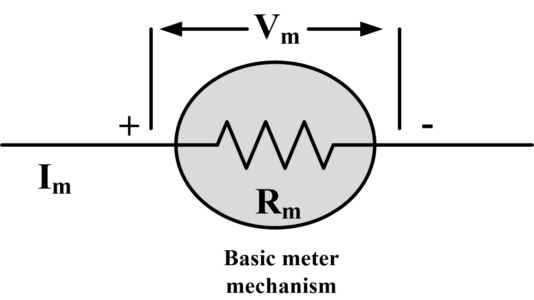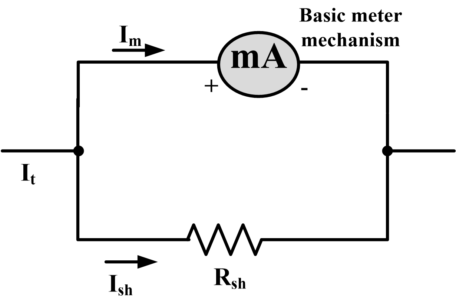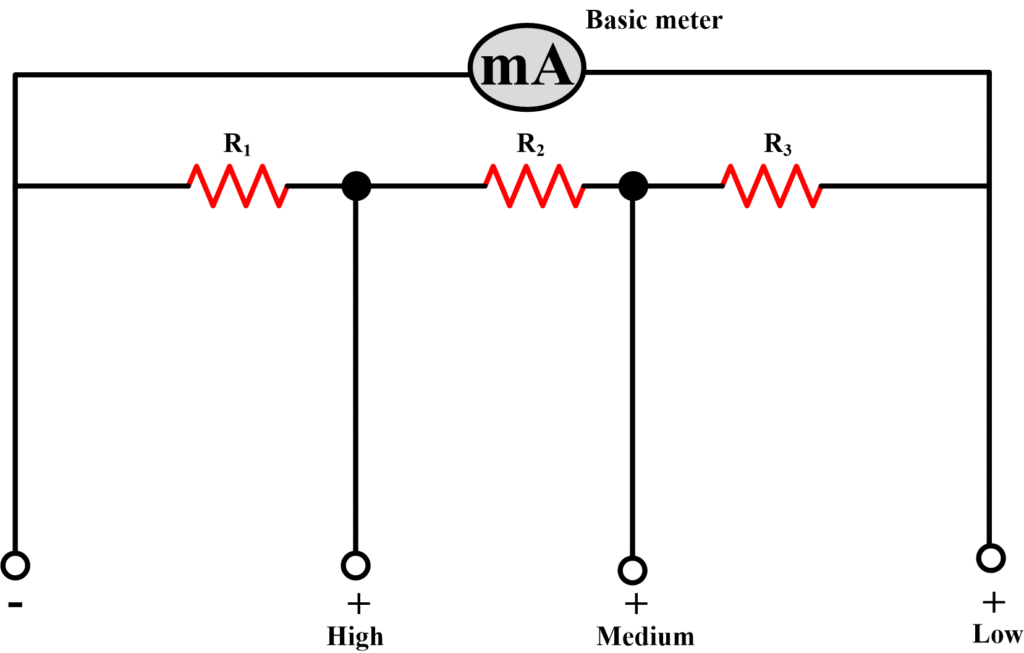Home / Instrumentation / Ammeter- Definition and Working Principle

# Ammeter- Definition and Working Principle

Want create site? Find Free WordPress Themes and plugins.

An Ammeter is a device used for the measurement of current in amperes.

When the current in a circuit is substantially less than an ampere, its value is determined by the use of a milliammeter or micro-ammeter, which measures full-scale currents in milli-amperes or microamperes, respectively. The symbol for the ammeter is a circle with the enclosed letter A, as shown in the following figure.In the above figure, an ammeter is inserted into the path of a current, that is, in series, so as to carry the current being measured.

If an ammeter is of DC type, then it has polarity signs on it for ensuring appropriate connection for an upscale indication. In particular, conventional current flows into the positive terminal for an upscale meter deflection as shown in above figure.

If an ammeter is of an AC type, then the direction of current has no importance and no polarity marks are needed on the meter. But, the meter is still connected in series fashion with the circuit for which the current is being computed.

It is obvious that a coil used in a basic meter mechanism has a certain resistance, Rm, called meter resistance, as shown in Fig.By Ohm’s law, when a current Im, sufficient to produce full-scale deflection, flows through the mechanism, a voltage drop, Vm is produced across the meter mechanism.

${{V}_{m}}={{I}_{m}}{{R}_{m}}$

Example

A dc milliammeter has a full-scale rating of 1 mA and a meter resistance of 25 Ohm. What voltage is across the meter when maximum current flows in the meter?

Solution

${{V}_{m}}={{I}_{m}}{{R}_{m}}=\left( 1*{{10}^{-3}} \right)*\left( 25 \right)=25~mV$

In order to use a meter mechanism to measure the current larger than the rating of the mechanism, a large part of the current, being monitored, must be bypassed or shunted around the mechanism as shown in the following figure.The value of shunt resistance, Rsh, needed to increase the current range, is calculated by using the full-scale value. With reference to above figure, if a meter with a full-scale rating of  is to indicate a full-scale deflection for a current  (>), then the shunted value of current is

${{I}_{sh}}={{I}_{t}}-{{I}_{m}}$

Since the shunt and meter form a parallel circuit, the voltage across both are equal and in terms of the resistance.

Example

It is desired to use the meter mechanism of above-mentioned example (1mA, 25 Ohm) to measure 50 mA at full scale. What shunt resistance is needed?

Solution

${{I}_{sh}}=50-1=49~mA$

${{I}_{sh}}{{R}_{sh}}={{I}_{m}}{{R}_{m}}=25~mV$

Therefore

${{R}_{sh}}=\frac{25*{{10}^{-3}}}{49*{{10}^{-3}}}=0.51~\Omega$

Multiple scale ammeters are constructed either by using switch arrangement or multiple terminals.The ammeter in above figure has a shunt made of series resistances. In the selection of progressive higher current ranges, more of the shunt resistance is shorted by the switch. The required resistances are calculated by first evaluating the resistance for the highest range and then progressing to each lower range to find what additional resistance is needed.The ammeter shown above, with multiple terminals is known as Ayrton shunt. In operating this type of ammeter, one uses the positive terminal and appropriate negative terminal (in the case of DC). This arrangement avoids the use of the switch and its variable contact resistance.

Did you find apk for android? You can find new Free Android Games and apps.

### About Ahmad FaizanMr. Ahmed Faizan Sheikh, M.Sc. (USA), Research Fellow (USA), a member of IEEE & CIGRE, is a Fulbright Alumnus and earned his Master’s Degree in Electrical and Power Engineering from Kansas State University, USA.